# Pokaznikiv-exponential equations

Definition: Pokaznikiv-exponential equation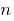— an equation that contains the expression type, i.e. equations of the form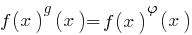## Main ways solution pokaznikiv-exponential equations and inequalities

### Example 1

Solutions: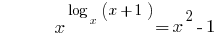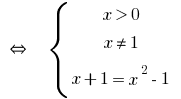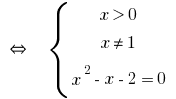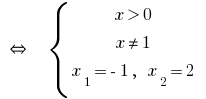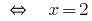Answer: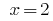### Example 2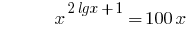Solutions:

At DHS,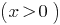both parts of the equation are positive, therefore, after taking the logarithm of base 10, we obtain the equation equivalent to this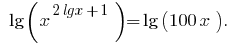Here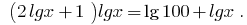Replacement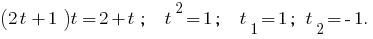Then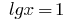or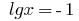, ie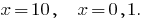(both the root lead to DHS)

Answer:### Example 3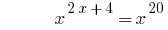Solutions:

If you count the basenumber, then

1)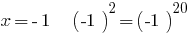the right to equality;

2) when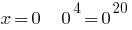properly;

3) when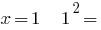properly;

4) if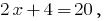that is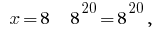true equality.

Answer: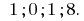Note: If count-basedvariable, the function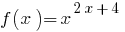is deemed to have arisen only when. From this point of view, this equation has only two roots: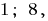Tags:
Chapter:
Versions in other languages:
Share with friends: